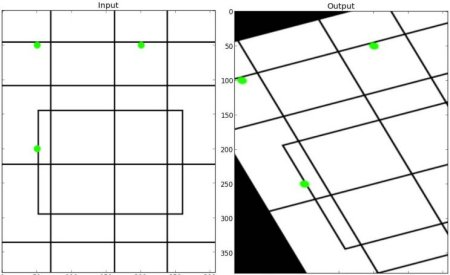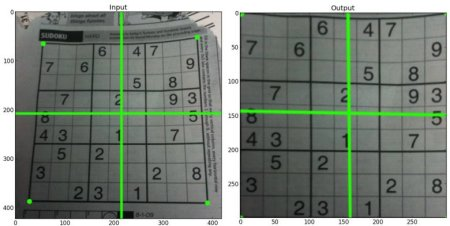# 【OpenCv-Python】十一、Geometric Transformations of Images 图像的几何变换

## 图像的几何变换

### 目标

• 在本教程中，你将学习几种简单变换。如：移动、旋转、仿射变换。
• 你将学习这些函数：cv2.getPerspectiveTransform等。

### 变换

openCv 提供了两种变换函数，cv2.warpAffine 和 cv2.warpPerspective。通过这两个函数，你可以实现所有的图像变换。cv2.warpAffine接受的参数是2x3的变换矩阵。cv2.warpPerspective 接受的参数是3x3的变换矩阵。

### 扩展缩放

import cv2
import numpy as np

res = cv2.resize(img,None,fx=2, fy=2, interpolation = cv2.INTER_CUBIC)

#或者

height, width = img.shape[:2]
res = cv2.resize(img,(2*width, 2*height), interpolation = cv2.INTER_CUBIC)

while(1):
cv2.imshow('res',res)
cv2.imshow('img',img)
if cv2.waitKey(1) & 0xFF == 27:
break
cv2.destroyAllWindows()


### 平移

import cv2
import numpy as np

rows,cols = img.shape

M = np.float32([[1,0,100],[0,1,50]])
dst = cv2.warpAffine(img,M,(cols,rows))

cv2.imshow('img',dst)
cv2.waitKey(0)
cv2.destroyAllWindows()


### 旋转

img = cv2.imread('messi5.jpg',0)
rows,cols = img.shape

M = cv2.getRotationMatrix2D((cols/2,rows/2),90,1)
dst = cv2.warpAffine(img,M,(cols,rows))### 仿射变换

img = cv2.imread('drawing.png')
rows,cols,ch = img.shape

pts1 = np.float32([[50,50],[200,50],[50,200]])
pts2 = np.float32([[10,100],[200,50],[100,250]])

M = cv2.getAffineTransform(pts1,pts2)

dst = cv2.warpAffine(img,M,(cols,rows))

plt.subplot(121),plt.imshow(img),plt.title('Input')
plt.subplot(122),plt.imshow(dst),plt.title('Output')
plt.show()### 透视变换



rows,cols,ch = img.shape

pts1 = np.float32([[56,65],[368,52],[28,387],[389,390]])
pts2 = np.float32([[0,0],[300,0],[0,300],[300,300]])

M = cv2.getPerspectiveTransform(pts1,pts2)

dst = cv2.warpPerspective(img,M,(300,300))

plt.subplot(121),plt.imshow(img),plt.title('Input')
plt.subplot(122),plt.imshow(dst),plt.title('Output')
plt.show()03-22247312-05773
05-15483
10-2929
11-20138
04-09692
09-26640
06-1190
02-251105
11-28273
11-02510
08-20
01-241万+
08-2310万+